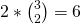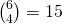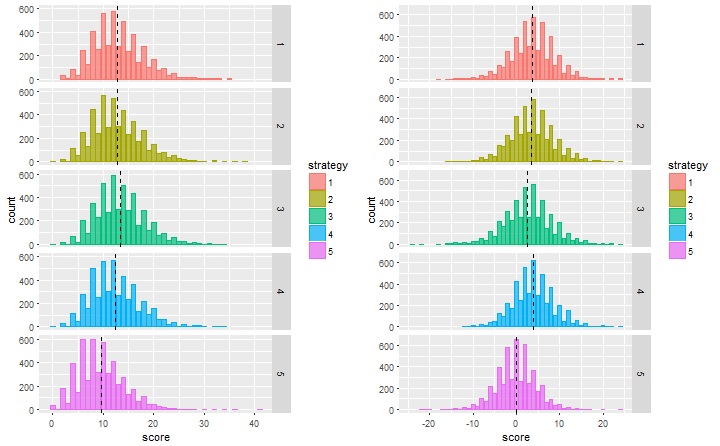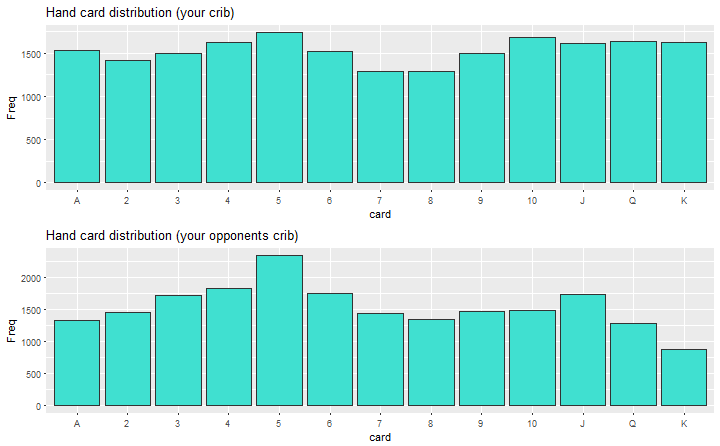Want to share your content on R-bloggers? click here if you have a blog, or here if you don't.Cribbage is one of my favourite card games and have been playing it ever since I could count. It is a unique card game, in fact it is often said there are 3 types of card games, trick style games, rummy style games and cribbage!

What I aim to do in a series of posts is take a data science approach to the game. I will simulate the game and eventually train an agent using a reinforcment learning model to play the game. Along the way I’ll simulate possible strategies such as keeping the highest scoring hand, keeping the best pegging hand, etc. This first post will simulate the deal for a 2 player game and choose the best 2 cards to throw into the crib to maximise your hand. Given how the game is played this may not actually be the best strategy, but before I get into that I’ll quickly explain the rules.

## The rules

The objective of the game is to be the first player to reach 121 points. Once a player reaches 121 the game ends immediately. There are several ways to get points,

• 2 points per unique combination of cards that sum to 15
• 2 points per unique pairs e.g. 2 Jacks = 2 points, 3 Jacks = 6 points ()
• 1 point per card for runs of 3 or more e.g. A, 2, 3
• 1 point per card for a flush of 4 or more cards (at least 4 cards need to be in your hand)
• 1 point for knobs i.e. a Jack in your hand the same suit as the card on the deck

Picture cards are all value of 10 and aces are low. There are more unique ways to pick up points which will be explained in one round.

1. The dealer deals 6 cards to each player.
2. Each player chooses 2 cards to throw into the crib. The crib is a second hand which is scored by the dealer at the end of the round.
3. The non-dealer cuts the deck and the dealer turns the top most card face up and places it on the deck. This counts as a 5th card for scoring purposes.
4. Starting with the non-dealer, each player plays a card keeping count of the cumulative score. If a player plays a card which sums to 15, creates a pair, or a run, they gain points accordingly. The cumulative score must not excede 31. The player to play the final card which ends on 31 gains 2 points. If a player cannot play a card they say “go”. Each player must play a card if they can. The last player to play a card gains 1 point. This continues until each player has played all their cards. Scoring points in this play is often called “pegging”.
5. Each player reveals their hand. Starting with the non-dealer (their first take) scores their hand (including the face up card on the deck) and moves their peg accordingly on the game track. Finally the dealer scores their hand.
6. The dealer reveals the crib and adds the score to their score.
7. The dealer swaps and 1-6 repeats.

Other random rules:

• You can only score a flush in the crib when there are 5 cards of the same suit.
• If the dealer turns over a Jack during the cut, they get 2 points for doing it.

## Strategy simulation

Here we will look at 5 possible strategies to begin with:

1. Expected maximum score for the hand
2. Highest potential score for the hand
3. Maximising both the hand and crib (when it’s your crib)
4. Maximising the hand and minimising the crib (when it’s your opponents crib)
5. Random selection for control

It is expected that strategy 5 will be the worst by far but it’s good to have a baseline. In many cases strategy 1 and 2 would end up choosing the same hand however there will be cases where they will suggest different hands. Strategy 3 may show an edge over the first 2. It could pick up on certain rules of thumb such as not breaking a pair or fifteen. Strategy 1 will often have multiple choices for the the maximum possible score. In this case the algorithm will choose which of those has the highest expected score.

Another consideration is when discarding cards into the crib, if it is your crib you’ll also want to maximise the score but if it’s your opponents you’ll want to minimise the score. These will be slightly different objective functions. We should see the performance of these strategies between strategies 3 and 4.

The easiest way to select the optimal hand given the strategy is through brute force and calculate the score for every possible combination of 4 out of 6 cards to keep in your hand (), and the cut card. Calculating the crib score is more challenging since there are more combinations given the other source of randomness of your opponent throwing cards into the crib.

### Cribbage scoring functions

The below functions will score any given hand including the cut card.

```# deal
deck <- paste0(sort(c(rep("A", 4), rep(2:10, 4), rep(c("J", "Q", "K"), 4))), rep(c("C", "D", "H", "S"), 13))
deal <- function(n = 6, hands = 1){
dealt <- sample(deck, n*hands)
hands <- lapply(1:hands, function(x) dealt[match.fun("==")(1:(n*hands) %% hands, x %% hands)])
return(hands)
}

# draw the cut card
cut <- function(hands) sample(deck[!(deck %in% unlist(hands))], 1)

# transformations
number <- function(hand) ifelse(nchar(hand) == 2, str_sub(hand, 1, 1), str_sub(hand, 1, 2))
suit <- function(hand) ifelse(nchar(hand) == 2, str_sub(hand, 2, 2), str_sub(hand, 3, 3))
extract.value <- function(x) {
if(x == "A"){
return(1)
}else if(x %in% c("J", "Q", "K")){
return(10)
}else{
return(as.numeric(x))
}
}
value <- function(hand)  return(unname(sapply(number(hand), extract.value)))

# representing the number of the card as an acutal number for the sake of calculating runs
convert.number <- function(x) {
if(x == "A"){
return(1)
}else if(x == "J"){
return(11)
}else if(x == "Q"){
return(12)
}else if(x == "K"){
return(13)
}else{
return(as.numeric(x))
}
}
card.to.num <- function(hand) unname(sapply(number(hand), convert.number))

# fifteens
fifteen <- function(hand){
hand.val <- value(hand)
score <- 0
for(k in 2:length(hand)){
combination.mat <- combn(hand.val, k)
n15 <- apply(combination.mat, 2, sum) == 15
score <- score + sum(n15)*2
}
return(score)
}

# pairs
pairs <- function(hand){
hand.num <- number(hand)
pair.vec <- apply(combn(hand.num, 2), 2, function(x) all(x == x))
return(sum(pair.vec)*2)
}

# runs
check.for.run <- function(x) all(x - x == seq(0, length(x)-1 , 1))
runs <- function(hand){
hand.num <- card.to.num(hand)
score <- 0
for(k in length(hand):3){
sort.comb.mat <- apply(combn(hand.num, k), 2, sort)
runk <- apply(sort.comb.mat, 2, check.for.run)
score <- sum(runk)*k
if(score > 0) break
}
return(score)
}

# flush
flush <- function(hand, cut, crib = FALSE){
hs <- suit(hand)
hcs <- suit(c(hand, cut))
if(all(hcs == hcs)){
return(5)
}else if(all(hs == hcs) & !crib){
return(4)
}else{
return(0)
}
}

# knobs
check.for.knobs <- function(x, cut) number(x) == "J" & suit(cut) == suit(x)
knobs <- function(hand, cut) sum(sapply(hand, function(x) check.for.knobs(x, cut)))

# calculate total score
total.score <- function(hand, cut, crib = FALSE)
fifteen(c(hand, cut)) +
pairs(c(hand, cut)) +
runs(c(hand, cut)) +
knobs(hand, cut) +
flush(hand, cut, crib)```

A few hands will be passed to the total.score() function just to ensure it is calculating the score correctly.

`total.score(hand = c("4S", "5C", "5S", "6H"), cut = "6D") # 24 - runs, pairs, 15's`

`##  24`

`total.score(hand = c("JS", "5C", "7S", "6H"), cut = "10S") # 8 - runs, 15's, knobs`

`##  8`

`total.score(hand = c("JS", "5C", "5D", "5H"), cut = "5S") # 29 - the mythical maximum hand`

`##  29`

`total.score(hand = c("9S", "8C", "8S", "8H"), cut = "10S") # 15 triple run`

`##  15`

`total.score(hand = c("9S", "8S", "AS", "2S"), cut = "KC", crib = FALSE) # flush in the hand`

`##  4`

`total.score(hand = c("9S", "8S", "AS", "2S"), cut = "KC", crib = TRUE) # almost flush in the crib`

`##  0`

All hands check out.

### Expected score

For any given hand the expected score and maximum possible score will be calculated for each combination of 4 cards kept in the hand. With regards to the crib we only know the 2 cards we are putting into the crib and the remaining 2 cards plus the cut card are effectively random. In practice this isn’t entirely the case though because your opponent is likely to throw out cards which limit the score gaining potential in the crib, as you would do if it was your opponents crib. Even with this simulation if we had two identical agents playing off against each other and each agents strategy was to maximise points in their hand, this would alter probability distribution for the cards to be discarded to the crib, for example 5’s are very valuable because there are lots of cards with a value of 10 in the deck so they are less likely to be in the crib. These probabilities will be estimated through an iterative process.

Given we only have knowledge of 2 cards out of the 5, scores will need to be calculated forpotential crib hands. To speed up the processing a master look up table will be created for every possible hand rather than computing 45k scores every simulation.

```# master list
# this took around 2.5 hours so not run here
no_cores <- detectCores()

n <- choose(52, 4)
hand.combn <- combn(deck, 4)
cut.combn <- apply(hand.combn, 2, function(x) deck[!(deck %in% x)])

strt <- Sys.time()
registerDoParallel(makeCluster(no_cores))
crib.master.table <- foreach(k = 1:n, .combine = rbind, .packages = c("stringr"))  %dopar%
unname(sapply(cut.combn[,k], total.score, hand = hand.combn[,k]))
stopImplicitCluster()
Sys.time() - strt```

The optimal hand function will select the best hand for each of the 5 strategies. It will output the key metrics for it’s decision such as the expected score, maximum score and expected crib score. This calculation will be relatively slow. There are many ways we could optimise the computation time, for example many hands will be the same since suit doesn’t factor into the score and therefore only need to compute the expected values for these hands once, although some times suit does play a roll. That becomes a more complex calculation so for now this will do. I’ll include the code for you to replicate/improve if you wish.

```# optimal hand function
optimal.hand <- function(hand, compute.crib = FALSE, strategy = NULL){

# given the hand compute the combinations of ways to keep 4 cards and throw 2 in the crib
remaining.in.deck <- deck[!(deck %in% unlist(hand))]
possible.hands <- combn(hand, 4)
crib.cards <- apply(possible.hands, 2, function(x) hand[!(hand %in% x)])
score.mat <- matrix(NA, nrow = length(remaining.in.deck), ncol = ncol(possible.hands))
best.hand <- list()
best.crib.cards <- list()
hand.combn <- combn(deck, 4)

# the random strategy
best.hand[] <- possible.hands[,sample(1:ncol(possible.hands), 1)]
best.crib.cards[] <- hand[!(hand %in% best.hand[])]

# break if random strat
if(!is.null(strategy)) if(strategy == 5) break

# compute the score for each hand combination and cut card
for(k in 1:ncol(possible.hands)) score.mat[,k] <- unname(sapply(remaining.in.deck, total.score, hand = possible.hands[,k]))
rownames(score.mat) <- remaining.in.deck

# compute crib for each combination of 2 cards discarded and cut card
# to speed up computation these are looked up in a master table
expected.crib.score <- NULL
if(compute.crib){
id <- apply(hand.combn, 2, function(x) sum(hand %in% x) > 1)
reduced.hand.combn <- hand.combn[,id]
reduced.crib.master.table <- crib.master.table[id,]
expected.crib.score <- rep(NA, nrow(possible.hands))
for(k in 1:ncol(possible.hands)){
possible.crib.hands <- apply(reduced.hand.combn, 2, function(x) all(crib.cards[,k] %in% x & !(possible.hands[,k] %in% x)))
expected.crib.score[k] <- mean(reduced.crib.master.table[possible.crib.hands,])
}
}

# compute the max and expected hands
max.hand <- apply(score.mat, 2, max)
expected.score <- apply(score.mat, 2, mean)

# select the best hand given strategy
# highest expected value
best.hand[] <- possible.hands[,which.max(expected.score)]
best.crib.cards[] <- hand[!(hand %in% best.hand[])]

# highest potential maximum
a <- which.max(as.numeric(max.hand == max(max.hand))*expected.score)
best.hand[] <- possible.hands[,a]
best.crib.cards[] <- hand[!(hand %in% best.hand[])]

# maximum expected score hand + crib
best.hand[] <- possible.hands[,which.max(expected.score+expected.crib.score)]
best.crib.cards[] <- hand[!(hand %in% best.hand[])]

# maximum expected hand lowest expected crib
best.hand[] <- possible.hands[,which.max(expected.score-expected.crib.score)]
best.crib.cards[] <- hand[!(hand %in% best.hand[])]

# select strat
if(is.null(strategy)) strategy <- 1:5

# return results
return(list(strategy = strategy,
hand = hand,
max = max.hand,
expected.score = expected.score,
expected.crib.score = expected.crib.score,
best.hand = best.hand[strategy],
crib.cards = best.crib.cards[strategy]
))
}```

```strt <- Sys.time()
x.score <- optimal.hand(c("KS", "4H", "8S", "9S", "5S", "3D"), compute.crib = TRUE, strategy = 3)
Sys.time() - strt```

`## Time difference of 4.731728 secs`

`x.score`

```## \$strategy
##  3
##
## \$hand
##  "KS" "4H" "8S" "9S" "5S" "3D"
##
## \$max
##    5  7  7  9  7 12 12  5  7  6  7  8 14 12  7
##
## \$expected.score
##   2.000000 4.586957 4.021739 4.630435 2.065217 8.021739 8.717391
##   1.956522 4.326087 4.326087 3.326087 4.543478 8.152174 6.195652
##  3.021739
##
## \$expected.crib.score
##   6.465761 4.153482 5.974497 4.266043 5.995632 5.229549 5.454610
##   7.075181 4.227234 4.257830 3.915076 7.091244 3.610588 3.668277
##  3.887701
##
## \$best.hand
## \$best.hand[]
##  "KS" "8S" "9S" "5S"
##
##
## \$crib.cards
## \$crib.cards[]
##  "4H" "3D"```

For this hand using strategy 3 the expected score is 8.7. This hand is also a good example of where strategy 1 and 2 suggest different cards to discard into the crib.

## Simulation

We will now simulate 5000 hands and score each hand by the 5 strategies and output the results.

```# simulation took 4 hours
N <- 5000
rhands <- lapply(1:N, function(x) deal(6)[])
rcut <- lapply(rhands, cut)
strt <- Sys.time()
opt.hand <- mclapply(rhands, optimal.hand, compute.crib = TRUE)
Sys.time() - strt
scores <- matrix(NA, nrow = N, ncol = 5)
crib.scores <- matrix(NA, nrow = N, ncol = 5)
for(k in 1:N) {
scores[k,] <- sapply(1:5, function(x) total.score(opt.hand[[k]]\$best.hand[[x]], rcut[[k]]))
crib.scores[k,] <- sapply(1:5, function(x) total.score(c(opt.hand[[k]]\$crib.cards[[x]], sample(deck[!(deck %in% c(rhands[[k]], rcut[[k]]))], 2)), rcut[[k]]))
}```

```df.your.crib <- melt(scores + crib.scores)
df.opp.crib <- melt(scores - crib.scores)
colnames(df.your.crib) <- c("sim", "strategy", "score")
colnames(df.opp.crib) <- c("sim", "strategy", "score")
df.your.crib\$strategy <- as.factor(df.your.crib\$strategy)
df.opp.crib\$strategy <- as.factor(df.opp.crib\$strategy)

# summary of results
res1 <- apply(scores + crib.scores, 2, summary); res1```

```##            [,1]    [,2]    [,3]    [,4]    [,5]
## Min.     2.0000  0.0000  0.0000  0.0000  0.0000
## 1st Qu. 10.0000  9.0000 10.0000  9.0000  6.0000
## Median  12.0000 12.0000 13.0000 12.0000  9.0000
## Mean    12.8894 12.7584 13.3572 12.3868  9.5798
## 3rd Qu. 16.0000 16.0000 16.0000 15.0000 12.0000
## Max.    35.0000 38.0000 34.0000 34.0000 41.0000```

`res2 <- apply(scores - crib.scores, 2, summary); res2`

```##             [,1]     [,2]     [,3]     [,4]     [,5]
## Min.    -18.0000 -16.0000 -24.0000 -12.0000 -22.0000
## 1st Qu.   0.0000   0.0000  -1.0000   1.0000  -3.0000
## Median    4.0000   4.0000   3.0000   4.0000   0.0000
## Mean      3.7394   3.5552   2.6456   3.8844   0.0258
## 3rd Qu.   7.0000   7.0000   6.0000   7.0000   3.0000
## Max.     24.0000  24.0000  24.0000  24.0000  24.0000```

```# means
score.means1 <- data.frame(strategy = levels(df.your.crib\$strategy), score.means = res1[4,])
score.means2 <- data.frame(strategy = levels(df.your.crib\$strategy), score.means = res2[4,])

# plots
grid.arrange(
ggplot(df.your.crib, aes(x = score, fill = strategy, col = strategy)) +
geom_bar(stat = "count", alpha = I(0.7)) +
geom_vline(aes(xintercept=score.means), data=score.means1, lty = 2) +
facet_grid(strategy ~ .),
ggplot(df.opp.crib, aes(x = score, fill = strategy, col = strategy)) +
geom_bar(stat = "count", alpha = I(0.7)) +
geom_vline(aes(xintercept=score.means), data=score.means2, lty = 2) +
facet_grid(strategy ~ .),
nrow = 1)```The random strategy is as expected the worst strategy which is clear to see in the summary table and the histograms, The mean score is 3-4 points lower than the others. There is less of a difference between the other 4 srategies however the table shows that strategy 3 will on average give the best score when it is your crib, and strategy 4 will when it’s your opponents crib. While it’s not much of an edge it could mean domination over 25 years of playing cribbage! But it’s also good to see the maths and logic checks out.

The distribution of cards that were chosen to remain in the hand for strategies 3 and 4 shows when it’s your crib 7’s and 8’s are the cards more likely to be thrown into the crib. Otherwise it’s a relatively uniform distribution for the other cards. When it’s your opponents crib it’s quite clear it’s better to keep 5’s (which makes perfect sense) and throw out K’s.

```strat3.hand <- data.frame(table(number(unlist(lapply(opt.hand, function(x) x\$best.hand[])))))
strat4.hand <- data.frame(table(number(unlist(lapply(opt.hand, function(x) x\$best.hand[])))))
colnames(strat3.hand) <- "card"
colnames(strat4.hand) <- "card"
strat3.hand\$card <- factor(strat3.hand\$card, levels = strat3.hand\$card[c(10, 2:9, 1, 11, 13, 12)])
strat4.hand\$card <- factor(strat4.hand\$card, levels = strat4.hand\$card[c(10, 2:9, 1, 11, 13, 12)])

grid.arrange(
ggplot(strat3.hand, aes(x = card, y = Freq)) +
geom_bar(stat = "identity", col = "grey20", fill = "turquoise") +
labs(title = "Hand card distribution (your crib)"),
ggplot(strat4.hand, aes(x = card, y = Freq)) +
geom_bar(stat = "identity", col = "grey20", fill = "turquoise") +
labs(title = "Hand card distribution (your opponents crib)")
)```This is strengthened by the distribution of cards thrown into the crib. When it’s your crib it’s best to throw out 7 and 8’s, and 2 and 3’s. When it’s your opponents crib it is best to throw out Kings and Queens. And there are very few cases where it is better for you to throw out a 5, which again makes sense.

```strat3.hand <- data.frame(table(number(unlist(lapply(opt.hand, function(x) x\$crib.cards[])))))
strat4.hand <- data.frame(table(number(unlist(lapply(opt.hand, function(x) x\$crib.cards[])))))
colnames(strat3.hand) <- "card"
colnames(strat4.hand) <- "card"
strat3.hand\$card <- factor(strat3.hand\$card, levels = strat3.hand\$card[c(10, 2:9, 1, 11, 13, 12)])
strat4.hand\$card <- factor(strat4.hand\$card, levels = strat4.hand\$card[c(10, 2:9, 1, 11, 13, 12)])

grid.arrange(
ggplot(strat3.hand, aes(x = card, y = Freq)) +
geom_bar(stat = "identity", col = "grey20", fill = "turquoise") +
labs(title = "Crib card distribution (your crib)"),
ggplot(strat4.hand, aes(x = card, y = Freq)) +
geom_bar(stat = "identity", col = "grey20", fill = "turquoise") +
labs(title = "Crib card distribution (your opponents crib)")
)```The optimal hands where simulated by assuming that the cards thrown into the crib were random. It is clear that if you opponent was using a better strategy the cards expected in the crib are very different. We can now re-score the optimal hands by using the above estimated probability distribution. This will again generate a different probability distribution. This is repeated until it converges on the maximum likelihood estimates.

## Final thoughts; Future posts

For this phase of the game it is clear strategy 3 and 4 will work out best but this is only part of the game. The “play” phase of the game is where a lot of points can be gained. There are cards which are better for scoring in the play phase or what is often called “pegging”. This is much harder to do via brute force since it depends on which cards your opponent was dealt, which ones they kept and in which order they played them. We’ll have to employ machine learing techniques to optimise which 4 cards to keep to maximise the score over the full play of the hand assuming the cards are played in the optimal order.

This is just the beggining of this analysis. In future posts I will

1. Improve on expected crib score by using the probability distributions of an opponent using strategy 3 or 4
2. Simulate the play phase of the game
3. Simulate the entire game
4. Fit a reinforcement learning model to train an agent to play crib. This will be the most intersting part of the project and given the game is played in two unique phases but are dependent on the initial deal and card selection, it will be a challenging exercise.
5. And just for fun – build an image recognition algorithm so you can take a photo of your hand and it will output the best selection!

Stay tuned.

The post Cribbage: Optimal Hand (part 1) appeared first on Daniel Oehm | Gradient Descending.